International
Tables for
Crystallography
Volume B
Reciprocal space
Edited by U. Shmueli

International Tables for Crystallography (2006). Vol. B. ch. 2.1, pp. 204-205   | 1 | 2 |

## Section 2.1.8.2. Fourier–Bessel series

U. Shmuelia* and A. J. C. Wilsonb

aSchool of Chemistry, Tel Aviv University, Tel Aviv 69 978, Israel, and bSt John's College, Cambridge, England
Correspondence e-mail:  ushmueli@post.tau.ac.il

#### 2.1.8.2. Fourier–Bessel series

| top | pdf |

Equations (2.1.8.5)and (2.1.8.9)are the exact counterparts of equations (2.1.7.5)and (2.1.7.6), respectively. The computational effort required to evaluate equation (2.1.8.9)is somewhat greater than that for (2.1.8.5), because a double Fourier series has to be summed. The p.d.f. for any noncentrosymmetric space group can be expressed by a double Fourier series, but this can be simplified if the characteristic function depends on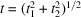alone, rather than on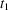and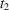separately. In such cases the p.d.f. offor a noncentrosymmetric space group can be expanded in a single Fourier–Bessel series (Barakat, 1974; Weiss & Kiefer, 1983; Shmueli et al., 1984). The general form of this expansion is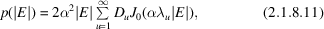where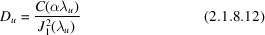and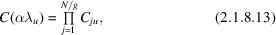where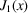is the Bessel function of the first kind, and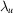is the uth root of the equation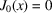; the atomic contribution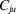to equation (2.1.8.13)is computed as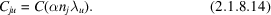The rootsare tabulated in the literature (e.g. Abramowitz & Stegun, 1972), but can be most conveniently computed as follows. The first five roots are given by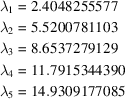and the higher ones can be obtained from McMahon's approximation (cf. Abramowitz & Stegun, 1972)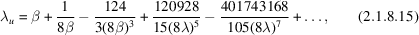where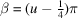. For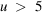the values given by equation (2.1.8.15)have a relative error less than 10−11 so that no refinement of roots of higher orders is needed (Shmueli et al., 1984). Numerical computations of single Fourier–Bessel series are of course faster than those of the double Fourier series, but both representations converge fairly rapidly.

### ReferencesAbramowitz, M. & Stegun, I. A. (1972). Handbook of mathematical functions. New York: Dover.Google ScholarBarakat, R. (1974). First-order statistics of combined random sinusoidal waves with application to laser speckle patterns. Opt. Acta, 21, 903–921.Google ScholarShmueli, U., Weiss, G. H., Kiefer, J. E. & Wilson, A. J. C. (1984). Exact random-walk models in crystallographic statistics. I. Space groupsand. Acta Cryst. A40, 651–660.Google ScholarWeiss, G. H. & Kiefer, J. E. (1983). The Pearson random walk with unequal step sizes. J. Phys. A, 16, 489–495.Google Scholar